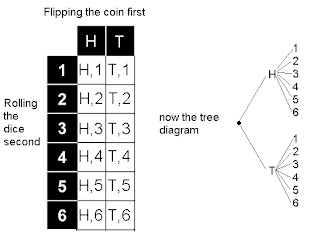## December 18, 2006

### Probability and math dictionary notes on it

Hey it's Ashley and I'm the scribe for today!
In the morning we started our new unit Probability. Mr.K said that Pascal invented Probability and its the same Pascal that did the Pascal's Triangle.
Mr.K asked us "What can happen if you roll six-sided die?"
Well you could get 6 possible answers. (i forgot what he said oops)

Soon we were asked "What can happen if you flip a coin twice?"
What you could do is draw a tree diagramNext question was a family of two children - "What is the probability they have one of each, a boy and a girl?"
Draw the tree diagram.That question and the flipping of the coin question are the same.

Next was "What if you roll a die twice?"
You could for the question draw a tree diagram but that would be too complicated so instead you could draw a table.Last question was "What if you flip a coin and roll a dice?"
Mr. K made us draw a table and a tree diagram.Towards the end of the period Mr.K handed us 2 booklets. One was yellow and one was green. Their both from the June 2005 exam. The title for this assignment is drum rolls please..... Go for Gold!!!

For this assignment you must:
- get 100% on it.
- complete the two booklets with no mistakes or errors.
- show all work including for the multiple choice questions either by writing a sentence explaining yourself but don't write a book. If there is no work shown or a sentence you get 0.
- must write in pencil! no pen or else you get 0.

In Go for Gold there are only two possible marks available. 0 and 100. It's worth 5% on our final class mark. Due on the Friday we get from winter break and you may get help from others and Mr.K.

In the afternoon Mr. K went over the questions on the quiz. If you want to see which ones you got right or wrong you could check now because it will show the answers. Mr.K got a question wrong on "Determine the foci of the hyperbola (y2/16) - (x2/25) = 1" by placing the answer on the wrong letter so that question won't count.

Next we took some notes down into our Math Dictionary

Probability

Probability: the branch of mathematics that deals with chance.
Sample Space (Ω): the set of all possible outcomes for a given "Experiment" represented by capital omega Ω.
Event (E): A subject of the sample space. A particular occurence in a given experiment.
Simple Event: The result of an experiment that is carried out in a single step.
Example: Flip a coin. The result is heads
(a simple event)
Compound Event: The result of an experiment carried out in two (or more) steps.
Example: 1. Flip a coin and roll a die - 6
The result is {H,6}
2. Flip a coin twice.
The result is {H,T}
Probability of an Event: (suppose to be sides to the box below but i don't know how to draw a rectangle so just put a box around it, i only managed the top and bottom borders)

_________________________________________________________
P(E) = number of favourable outcomes

Total number of possible outcomes (sample space) ________________________________________________________

Probability can be expressed as:
- a Ratio
- a Fraction
- a Decimal
- a Percent

(rectangle box below but i only got the top and bottom border, not the sides)
IMPORTANT: Probability of any event is always a number between 0 and _______________________________________________________________

Certain Event: an event whose probability is 1
example: roll a die - 6 and get a result less than 10
Impossible Event: an event whose probability is 0.
example: roll a die - 6 and get a 7
Complimentary Event: the compliment of E is E',
If P(E) = a then P(E') = 1-a
example: Given a standard deck of cards, a card is drawn at random.
P(spade) = (13/52) = (1/4) P (not a spade) = 1 - (1/4) = (3/4)

And that was the end of the math dictionary notes

Mr. K recommended we visit these sites:

math40s.com because it has the entire curriculum on it and some other stuff on it which I forgot but apparently it's a very good site.
webct.merlin.mb.ca because it too has the entire curriculum on it. you need to log on to this site.
it asks if you trust this site I think and you say yes.
both sites are good for reviewing.

** Don't forget to check the rubric for the Flickr assignment and leave your comments and what you like and what you want to change because the Rubric will be finalized on Friday. To leave a comment just press the Insert Comment button. The next Flickr assignment is on Exponential function which is due the Tuesday after winter break. The tag will be expfunc.
So that's it for my scribe.

Homework assignment is Ex.39 by the way.

Next scribe is........ well you know who you are *cough*Oliver*cough*

1.geeze...this is an awesome scribe post..it's so neat and precise and I don't think I've ever done this but I'm going to nominate your scribe for the hall of fame =)..good job Ashley.

2.I agree Ashley... you did an awesome job!! definitely hall of fame worthy!! ... nice pictures :D

3.Hi Ashley,

Your graphics really contributed to my understanding of your content! What an excellent scribe-- Hall of Fame worthy!

Best,
Lani